GeeksforGeeks App
Open AppBrowser
Continue

## Related Articles

• Write an Interview Experience
• Java Tutorial
• Java Collection Tutorial

# Java.lang.Integer class in Java

Integer class is a wrapper class for the primitive type int which contains several methods to effectively deal with an int value like converting it to a string representation, and vice-versa. An object of the Integer class can hold a single int value.

Constructors:

• Integer(int b): Creates an Integer object initialized with the value provided.

Syntax:

`public Integer(int b)`

Parameters:

`b : value with which to initialize`
• Integer(String s): Creates an Integer object initialized with the int value provided by string representation. Default radix is taken to be 10.

Syntax:

`public Integer(String s) throws NumberFormatException`

Parameters:

`s : string representation of the int value `

Throws:

```NumberFormatException :
If the string provided does not represent any int value.```

Methods:

1. toString() : Returns the string corresponding to the int value.

Syntax:

`public String toString(int b)`

Parameters :

`b : int value for which string representation required.`

2. toHexString() : Returns the string corresponding to the int value in hexadecimal form, that is it returns a string representing the int value in hex characters-[0-9][a-f]

Syntax:

`public String toHexString(int b)`

Parameters:

`b : int value for which hex string representation required.`

3. toOctalString() : Returns the string corresponding to the int value in octal form, that is it returns a string representing the int value in octal characters-[0-7]

Syntax:

`public String toOctalString(int b)`

Parameters:

`b : int value for which octal string representation required.`

4. toBinaryString() : Returns the string corresponding to the int value in binary digits, that is it returns a string representing the int value in hex characters-[0/1]

Syntax:

`public String toBinaryString(int b)`

Parameters:

`b : int value for which binary string representation required.`

5. valueOf() : returns the Integer object initialised with the value provided.

Syntax:

`public static Integer valueOf(int b)`

Parameters:

`b : a int value`

Syntax:

```public static Integer valueOf(String val, int radix)
throws NumberFormatException```

Parameters:

```val : String to be parsed into int value

Throws:

`NumberFormatException : if String cannot be parsed to a int value in given radix.`
• valueOf(String val): Another overloaded function which provides function similar to new Integer(Integer.parseInt(val,10))

Syntax:

```public static Integer valueOf(String s)
throws NumberFormatException```

Parameters:

`s : a String object to be parsed as int`

Throws:

`NumberFormatException : if String cannot be parsed to a int value in given radix.`

6. parseInt() : returns int value by parsing the string in radix provided. Differs from valueOf() as it returns a primitive int value and valueOf() return Integer object.

Syntax:

```public static int parseInt(String val, int radix)
throws NumberFormatException```

Parameters:

```val : String representation of int

Throws:

`NumberFormatException : if String cannot be parsed to a int value in given radix.`
• Another overloaded method containing only String as a parameter, radix is by default set to 10.

Syntax:

```public static int parseInt(String val)
throws NumberFormatException```

Parameters:

`val : String representation of int `

Throws:

`NumberFormatException : if String cannot be parsed to a int value in given radix.`

7. getInteger(): returns the Integer object representing the value associated with the given system property or null if it does not exist.

Syntax:

`public static Integer getInteger(String prop)`

Parameters :

`prop : System property`
• Another overloaded method which returns the second argument if the property does not exist, that is it does not return null but a default value supplied by user.

Syntax:

`public static Integer getInteger(String prop, int val)`

Parameters:

```prop : System property
val : value to return if property does not exist.```
• Another overloaded method which parses the value according to the value returned, that is if the value returned starts with “#”, than it is parsed as hexadecimal, if starts with “0”, than it is parsed as octal, else decimal.

Syntax:

`public static Integer getInteger(String prop, Integer val)`

Parameters:

```prop : System property
val : value to return if property does not exist.```

8. decode() : returns a Integer object holding the decoded value of string provided. String provided must be of the following form else NumberFormatException will be thrown-
Decimal- (Sign)Decimal_Number
Hex- (Sign)”0x”Hex_Digits
Hex- (Sign)”0X”Hex_Digits
Octal- (Sign)”0″Octal_Digits

Syntax:

```public static Integer decode(String s)
throws NumberFormatException```

Parameters:

`s : encoded string to be parsed into int val`

Throws:

`NumberFormatException : If the string cannot be decoded into a int value`

9. rotateLeft() : Returns a primitive int by rotating the bits left by given distance in two’s complement form of the value given. When rotating left, the most significant bit is moved to the right-hand side, or least significant position i.e. cyclic movement of bits takes place. Negative distance signifies right rotation.

Syntax:

`public static int rotateLeft(int val, int dist)`

Parameters:

```val : int value to be rotated
dist : distance to rotate```

10. rotateRight() : Returns a primitive int by rotating the bits right by given distance in the twos complement form of the value given. When rotating right, the least significant bit is moved to the left hand side, or most significant position i.e. cyclic movement of bits takes place. Negative distance signifies left rotation.

Syntax:

`public static int rotateRight(int val, int dist)`

Parameters:

```val : int value to be rotated
dist : distance to rotate```

## Java

 `// Java program to illustrate``// various Integer methods``public` `class` `Integer_test {``    ``public` `static` `void` `main(String args[])``    ``{``        ``int` `b = ``55``;``        ``String bb = ``"45"``;` `        ``// Construct two Integer objects``        ``Integer x = ``new` `Integer(b);``        ``Integer y = ``new` `Integer(bb);` `        ``// toString()``        ``System.out.println(``"toString(b) = "``                           ``+ Integer.toString(b));` `        ``// toHexString(),toOctalString(),toBinaryString()``        ``// converts into hexadecimal, octal and binary``        ``// forms.``        ``System.out.println(``"toHexString(b) ="``                           ``+ Integer.toHexString(b));``        ``System.out.println(``"toOctalString(b) ="``                           ``+ Integer.toOctalString(b));``        ``System.out.println(``"toBinaryString(b) ="``                           ``+ Integer.toBinaryString(b));` `        ``// valueOf(): return Integer object``        ``// an overloaded method takes radix as well.``        ``Integer z = Integer.valueOf(b);``        ``System.out.println(``"valueOf(b) = "` `+ z);``        ``z = Integer.valueOf(bb);``        ``System.out.println(``"ValueOf(bb) = "` `+ z);``        ``z = Integer.valueOf(bb, ``6``);``        ``System.out.println(``"ValueOf(bb,6) = "` `+ z);` `        ``// parseInt(): return primitive int value``        ``// an overloaded method takes radix as well``        ``int` `zz = Integer.parseInt(bb);``        ``System.out.println(``"parseInt(bb) = "` `+ zz);``        ``zz = Integer.parseInt(bb, ``6``);``        ``System.out.println(``"parseInt(bb,6) = "` `+ zz);` `        ``// getInteger(): can be used to retrieve``        ``// int value of system property``        ``int` `prop``            ``= Integer.getInteger(``"sun.arch.data.model"``);``        ``System.out.println(``            ``"getInteger(sun.arch.data.model) = "` `+ prop);``        ``System.out.println(``"getInteger(abcd) ="``                           ``+ Integer.getInteger(``"abcd"``));` `        ``// an overloaded getInteger() method``        ``// which return default value if property not found.``        ``System.out.println(``            ``"getInteger(abcd,10) ="``            ``+ Integer.getInteger(``"abcd"``, ``10``));` `        ``// decode() : decodes the hex,octal and decimal``        ``// string to corresponding int values.``        ``String decimal = ``"45"``;``        ``String octal = ``"005"``;``        ``String hex = ``"0x0f"``;` `        ``Integer dec = Integer.decode(decimal);``        ``System.out.println(``"decode(45) = "` `+ dec);``        ``dec = Integer.decode(octal);``        ``System.out.println(``"decode(005) = "` `+ dec);``        ``dec = Integer.decode(hex);``        ``System.out.println(``"decode(0x0f) = "` `+ dec);` `        ``// rotateLeft and rotateRight can be used``        ``// to rotate bits by specified distance``        ``int` `valrot = ``2``;``        ``System.out.println(``            ``"rotateLeft(0000 0000 0000 0010 , 2) ="``            ``+ Integer.rotateLeft(valrot, ``2``));``        ``System.out.println(``            ``"rotateRight(0000 0000 0000 0010,3) ="``            ``+ Integer.rotateRight(valrot, ``3``));``    ``}``}`

Output:

```toString(b) = 55
toHexString(b) =37
toOctalString(b) =67
toBinaryString(b) =110111
valueOf(b) = 55
ValueOf(bb) = 45
ValueOf(bb,6) = 29
parseInt(bb) = 45
parseInt(bb,6) = 29
getInteger(sun.arch.data.model) = 64
getInteger(abcd) =null
getInteger(abcd,10) =10
decode(45) = 45
decode(005) = 5
decode(0x0f) = 15
rotateLeft(0000 0000 0000 0010 , 2) =8
rotateRight(0000 0000 0000 0010,3) =1073741824```

11. byteValue() : returns a byte value corresponding to this Integer Object.

Syntax:

`public byte byteValue()`

12. shortValue() : returns a short value corresponding to this Integer Object.

Syntax:

`public short shortValue()`

13. intValue() : returns a int value corresponding to this Integer Object.

Syntax:

`public int intValue()`

13. longValue() : returns a long value corresponding to this Integer Object.

Syntax:

`public long longValue()`

14. doubleValue() : returns a double value corresponding to this Integer Object.

Syntax:

`public double doubleValue()`

15. floatValue() : returns a float value corresponding to this Integer Object.

Syntax:

`public float floatValue()`

16. hashCode() : returns the hashcode corresponding to this Integer Object.

Syntax:

`public int hashCode()`

17. bitcount() : Returns number of set bits in twos complement of the integer given.

Syntax:

`public static int bitCount(int i)`

Parameters:

`i : int value whose set bits to count`

18. numberOfLeadingZeroes() : Returns number of 0 bits preceding the highest 1 bit in twos complement form of the value, i.e. if the number in twos complement form is 0000 1010 0000 0000, then this function would return 4.

Syntax:

`public static int numberofLeadingZeroes(int i)`

Parameters:

`i : int value whose leading zeroes to count in twos complement form`

19. numberOfTrailingZeroes() : Returns number of 0 bits following the last 1 bit in twos complement form of the value, i.e. if the number in twos complement form is 0000 1010 0000 0000, then this function would return 9.

Syntax:

`public static int numberofTrailingZeroes(int i)`

Parameters:

`i : int value whose trailing zeroes to count in twos complement form`

20. highestOneBit() : Returns a value with at most a single one bit, in the position of highest one bit in the value given. Returns 0 if the value given is 0, that is if the number is 0000 0000 0000 1111, then this function return 0000 0000 0000 1000 (one at highest one bit in the given number)

Syntax:

`public static int highestOneBit(int i)`

Parameters:

`i : int value `

21. LowestOneBit() : Returns a value with at most a single one bit, in the position of lowest one bit in the value given. Returns 0 if the value given is 0, that is if the number is 0000 0000 0000 1111, then this function return 0000 0000 0000 0001 (one at highest one bit in the given number)

Syntax:

`public static int LowestOneBit(int i)`

Parameters:

`i : int value `

22. equals() : Used to compare the equality of two Integer objects. This method returns true if both the objects contain the same int value. Should be used only if checking for equality. In all other cases, the compareTo method should be preferred.

Syntax:

`public boolean equals(Object obj)`

Parameters:

`obj : object to compare with`

23. compareTo() : Used to compare two Integer objects for numerical equality. This should be used when comparing two Integer values for numerical equality as it would differentiate between less and greater values. Returns a value less than 0,0, a value greater than 0 for less than, equal to and greater than.

Syntax:

`public int compareTo(Integer b)`

Parameters:

`b : Integer object to compare with`

24. compare(): Used to compare two primitive int values for numerical equality. As it is a static method therefore it can be used without creating any object of Integer.

Syntax:

`public static int compare(int x,int y)`

Parameters:

```x : int value
y : another int value```

25. signum() : returns -1 for negative values, 0 for 0 and +1 for values greater than 0.

Syntax:

`public static int signum(int val)`

Parameters:

`val : int value for which signum is required.`

26. reverse(): returns a primitive int value reversing the order of bits in two’s complement form of the given int value.

Syntax:

`public static int reverseBytes(int val)`

Parameters:

`val : int value whose bits to reverse in order.`

27. reverseBytes() : returns a primitive int value reversing the order of bytes in two’s complement form of the given int value.

Syntax:

`public static int reverseBytes(int val)`

Parameters:

`val : int value whose bits to reverse in order.`

28. static int compareUnsigned(int x, int y): This method compares two int values numerically treating the values as unsigned.

Syntax:

`public static int compareUnsigned(int x, int y)`

29. static int divideUnsigned(int dividend, int divisor): This method returns the unsigned quotient of dividing the first argument by the second where each argument and the result is interpreted as an unsigned value.

Syntax:

`public static int divideUnsigned(int dividend, int divisor)`

30. static int max(int a, int b): This method returns the greater of two int values as if by calling Math.max.

Syntax:

`public static int max(int a, int b)`

31. static int min(int a, int b): This method returns the smaller of two int values as if by calling Math.min.

Syntax:

`public static int min(int a, int b)`

32. static int parseUnsignedInt(CharSequence s, int beginIndex, int endIndex, int radix): This method parses the CharSequence argument as an unsigned int in the specified radix, beginning at the specified beginIndex and extending to endIndex – 1.

Syntax:

```public static int parseUnsignedInt(CharSequence s,
int beginIndex,
int endIndex,
throws NumberFormatException```

33. static int parseUnsignedInt(String s): This method parses the string argument as an unsigned decimal integer.

Syntax:

```public static int parseUnsignedInt(String s)
throws NumberFormatException```

34. static int parseUnsignedInt(String s, int radix): This method parses the string argument as an unsigned integer in the radix specified by the second argument.

Syntax:

```public static int parseUnsignedInt(String s,
throws NumberFormatException```

35. static int remainderUnsigned(int dividend, int divisor): This method returns the unsigned remainder from dividing the first argument by the second where each argument and the result is interpreted as an unsigned value.

Syntax:

`public static int remainderUnsigned(int dividend, int divisor)`

36. static int sum(int a, int b): This method adds two integers together as per the + operator.

Syntax:

`public static int sum(int a, int b)`

37. static long toUnsignedLong(int x): This method converts the argument to a long by an unsigned conversion.

Syntax:

`public static long toUnsignedLong(int x)    `

38. static String toUnsignedString(int i): This method returns a string representation of the argument as an unsigned decimal value.

Syntax:

`public static String toUnsignedString(int i, int radix) `

## Java

 `// Java program to illustrate``// various Integer class methods``public` `class` `Integer_test {``    ``public` `static` `void` `main(String args[])``    ``{``        ``int` `b = ``55``;``        ``String bb = ``"45"``;` `        ``// Construct two Integer objects``        ``Integer x = ``new` `Integer(b);``        ``Integer y = ``new` `Integer(bb);` `        ``// xxxValue can be used to retrieve``        ``// xxx type value from int value.``        ``// xxx can be int,byte,short,long,double,float``        ``System.out.println(``"bytevalue(x) = "``                           ``+ x.byteValue());``        ``System.out.println(``"shortvalue(x) = "``                           ``+ x.shortValue());``        ``System.out.println(``"intvalue(x) = "` `+ x.intValue());``        ``System.out.println(``"longvalue(x) = "``                           ``+ x.longValue());``        ``System.out.println(``"doublevalue(x) = "``                           ``+ x.doubleValue());``        ``System.out.println(``"floatvalue(x) = "``                           ``+ x.floatValue());` `        ``int` `value = ``45``;` `        ``// bitcount() : can be used to count set bits``        ``// in twos complement form of the number``        ``System.out.println(``"Integer.bitcount(value)="``                           ``+ Integer.bitCount(value));` `        ``// numberOfTrailingZeroes and numberOfLeadingZeroes``        ``// can be used to count prefix and postfix sequence``        ``// of 0``        ``System.out.println(``            ``"Integer.numberOfTrailingZeros(value)="``            ``+ Integer.numberOfTrailingZeros(value));``        ``System.out.println(``            ``"Integer.numberOfLeadingZeros(value)="``            ``+ Integer.numberOfLeadingZeros(value));` `        ``// highestOneBit returns a value with one on highest``        ``// set bit position``        ``System.out.println(``"Integer.highestOneBit(value)="``                           ``+ Integer.highestOneBit(value));` `        ``// highestOneBit returns a value with one on lowest``        ``// set bit position``        ``System.out.println(``"Integer.lowestOneBit(value)="``                           ``+ Integer.lowestOneBit(value));` `        ``// reverse() can be used to reverse order of bits``        ``// reverseBytes() can be used to reverse order of``        ``// bytes``        ``System.out.println(``"Integer.reverse(value)="``                           ``+ Integer.reverse(value));``        ``System.out.println(``"Integer.reverseBytes(value)="``                           ``+ Integer.reverseBytes(value));` `        ``// signum() returns -1,0,1 for negative,0 and``        ``// positive values``        ``System.out.println(``"Integer.signum(value)="``                           ``+ Integer.signum(value));` `        ``// hashcode() returns hashcode of the object``        ``int` `hash = x.hashCode();``        ``System.out.println(``"hashcode(x) = "` `+ hash);` `        ``// equals returns boolean value representing``        ``// equality``        ``boolean` `eq = x.equals(y);``        ``System.out.println(``"x.equals(y) = "` `+ eq);` `        ``// compare() used for comparing two int values``        ``int` `e = Integer.compare(x, y);``        ``System.out.println(``"compare(x,y) = "` `+ e);` `        ``// compareTo() used for comparing this value with``        ``// some other value``        ``int` `f = x.compareTo(y);``        ``System.out.println(``"x.compareTo(y) = "` `+ f);``    ``}``}`

Output :

```bytevalue(x) = 55
shortvalue(x) = 55
intvalue(x) = 55
longvalue(x) = 55
doublevalue(x) = 55.0
floatvalue(x) = 55.0
Integer.bitcount(value)=4
Integer.numberOfTrailingZeros(value)=0
Integer.highestOneBit(value)=32
Integer.lowestOneBit(value)=1
Integer.reverse(value)=-1275068416
Integer.reverseBytes(value)=754974720
Integer.signum(value)=1
hashcode(x) = 55
x.equals(y) = false
compare(x,y) = 1
x.compareTo(y) = 1```

Initialization of Integer wrapper class in Java :

Type 1: Initializing directly:

A constant object of Integer class will be created inside the space of constants in the heap memory. Space of constants: It is just to imagine for better understanding that there is some space for constants in heap memory.

Example:

```Integer x = 200;  //initializing directly
x = 300;      //modifying x
x = 10;           //modifying x again```

Integer x = 200

• The compiler converts the above statement into: Integer x=Integer.valueOf(200) . This is known as “Autoboxing”. The primitive integer value 200 is converted into an object.

( To understand Autoboxing & Unboxing check here: https://www.geeksforgeeks.org/autoboxing-unboxing-java/ )

• x points to 200 which is present in the space of constants. Refer Fig. 1.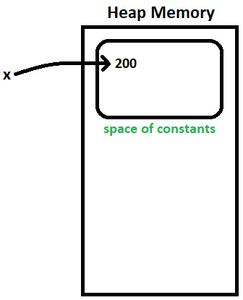Fig. 1

x = 300

• Autoboxing is done again because x is an Integer class object which is directly initialized.
• Note: The directly initialized object(x) cannot be modified as it is a constant. When we try to modify the object by pointing to a new constant(300), the old constant(200) will be present in heap memory, but the object will be pointing the new constant.
• x points to 300 which is present in the space of constants. Refer Fig. 2.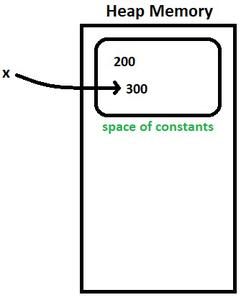Fig. 2

x = 10

• Note: By default for the values -128 to 127, Integer.valueOf() method will not create a new instance of Integer. It returns a value from its cache.
• x points 10 which is present in cache.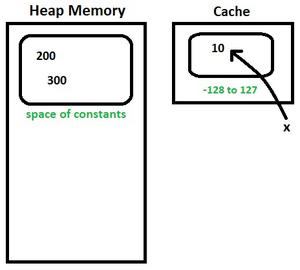Fig. 3

If we assign x = 200 or x=300 next time, it will point to the value 200 or 300 which is present already in space of constants. If we assign values to x other than these two values, then it creates a new constant.

(Check the Integer wrapper class comparison topic for better understanding)

Type 2: Initializing dynamically:

An Integer class object which is not a constant will be created outside the space of constants. It also creates a Integer constant inside the space of constants. The variable will be pointing to the Integer object & not the Integer constant.

Example:

```Integer a = new Integer(250);   //Initializing dynamically
a = 350;            //Type 1 initialization```

Integer a = new Integer(250)

• 250 is created inside and outside the space of constants. Variable ‘a’ will be pointing the value that is outside the space of constants. Refer Fig. 4.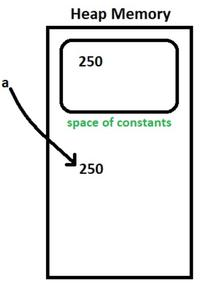Fig. 4

a = 350;

• After autoboxing, ‘a’ will be pointing to 350. Refer Fig. 5.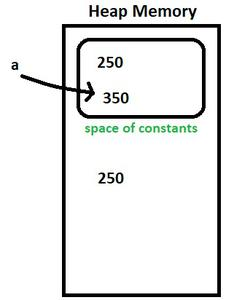Fig. 5

If we assign a = 250 next time, it will not point to the object already present with same value, it will create a new object.